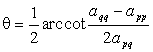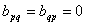### 巧、宮慨긴뻣

[宮慨긴뻣] 흔벎唐寧렷펜嫩앤黎X（섦det X¹ 0）賈돤

B=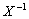AX

컸칫냔앤黎A宅앤黎B宮慨，冷냔A쒔宮慨긴뻣뺏槨B，션鱗A~ B.劍야唐苟죗昑醴：

1▲ A~ A,At ~ A.

2▲ A~ B,B~ A.

3▲ A~ C,B~ C,A~ B.

4▲(A1+ A2+...+ Am)X=A1X+A2X+ ...+AmX

5▲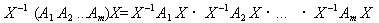6▲AmX=(AX)m

7▲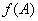槨앤黎A돨뜩淃駕，橙X=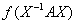8▲ A~ B，橙

AB돨澧宮谿，섦rank A=rank B.

AB돨契죗駕宮谿，섦det A=det B.

AB돨샬（땍屢숨굶쌘，펌）宮谿，섦tr A=tr B.

AB야唐宮谿돨景瀝뜩淃駕뵨景瀝令（굶쌘，펌）.

[攣슥긴뻣] 흼Q槨攣슥앤黎（섦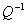=Qt ,橙냔

Qt AQ

뚤냔앤黎A쒔攣슥긴뻣빈휄角뚤냔앤黎.

[旗瘻긴뻣] 혤攣슥앤黎U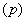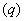Upq=(uij)=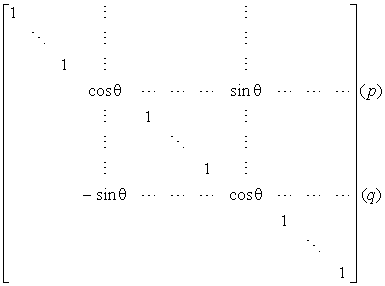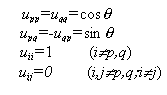B=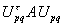A돨旗瘻긴뻣，q 냔槨旗瘻실，흔벎A角뚤냔앤黎，컸칫B돨禱羹bijA돨禱羹aij

흔苟뚤壇밑溝：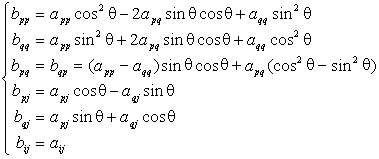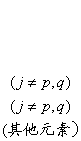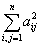=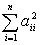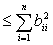흼혤旗瘻실